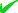News By Tag
Industry News
News By Place

Country(s)

Industry News

December 2021
SuSaFrThWeTuMo
54321
November 2021
3029

# Statistics Help - January 2019 Problem of the Month - Normal Distribution, Z-score

As a number of college students need help with normal distribution, z-score problems, TutorTeddy.com has come up with this exercise. Solving the following problem can help students gain a better understanding and resolve similar conceptual problems.

CAMBRIDGE, Mass. - Jan. 14, 2019 - PRLog -- The following exemplifies a general normal distribution problem normalized into a Z-score problem with usage of the Z-score table. Over 30% of the problems that Tutorteddy encounters are related to calculating Z-value.

Question:

Monthly Utility bills are normally distributed with a mean value of \$160 and standard deviation of \$25.
a. Find probability of bill between \$120 and \$180
b.Find probability of bill less than \$120
c.Find probability of bill more than \$210

Define X as the monthly utility bill and Z as a standard normal random variable.
(a) P(120 < X < 180) = P((120-160)/25 < Z < (180-160)/25) = 0.7333
(b) P(X < 120) = P(Z < (120-160)/25) = 0.0548
(c) P(X > 210) = P(Z > (210-160)/25) = 0.0228

To see more examples or receive help, visit https://tutorteddy.com/site/free_statistics_help.php. You can also get help with accounting problems at https://tutorteddy.com/free_accounting_homework_help.php.

Free help is available if needed for 15 minutes or less.
Tutorteddy.com is committed to spreading knowledge and encouraging learning, but we cannot help students unless they want to learn. Learn how to learn from TutorTeddy, and enjoy the challenge of life long growth.

Media Contact
Subhankar Ray
***@tutorteddy.com
6173958864
End
Email Tags : ***@tutorteddy.com : Statistics Help : Education : Cambridge - Massachusetts - United States : Services
Account Email AddressAccount Phone NumberDisclaimer     Report Abuse